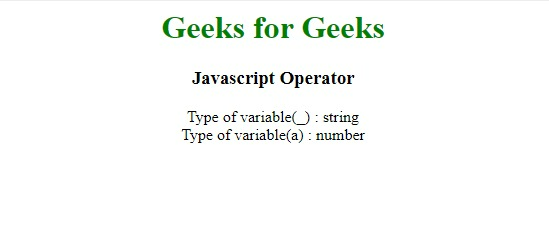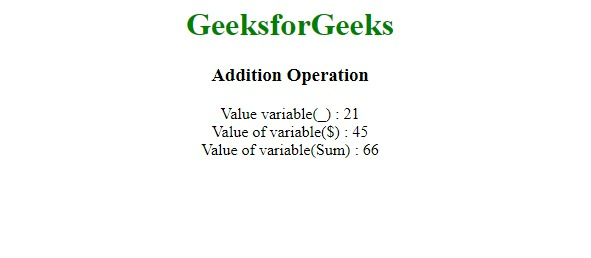GFG App
Open AppBrowser
Continue

# What does +_ operator mean in JavaScript ?

Unary Operator: A unary operation contain only one operand. Here, the ‘+’ unary plus operator converts its operand to Number type. While it also acts as an arithmetic operator with two operands which returns an addition result on calculation.

• JavaScript Identifiers: Javascript Identifiers are used to name variables (and keywords, functions, and labels). In Javascript, the first character must be a letter, or an underscore ( _ ), or a dollar sign ( \$ ) but not a digit and subsequent characters may be letters, digits, underscores, or dollar signs.
• JavaScript +_  operator: It is a combination of the variable with the symbol underscore( _ ) and unary plus ( + ) operator, + operator converts the type of _ variable to Number type for further operation.

Example: The variable “_” with string type is stored in variable “r” with “number” type.

Input:

```var _ = "1";
var r = +_;```

Output:

`typeof(r) will be number`

Example 1: Conversion of String to Number

## HTML

 `<``body` `style``=``"text-align:center"``> ` `    ``<``div` `class``=``"container"``> ` `        ``<``h1` `style``=``"color:green"``>Geeks for Geeks ` ` `  `        ``<``h3``> ` `            ``Javascript Operator ` `        `` ` ` `  `        ``<``p` `align``=``"center"``> ` `            ``Type of variable(_) : ` `            ``<``span` `id``=``"gfg"``> <``br` `/> ` `            ``Type of variable(a) : ` `            ``<``span` `id``=``"gfg1"``> ` `        `` ` ` `  `        ``<``script` `type``=``"text/javascript"``> ` `            ``GFG = function (_) { ` `                ``let b = typeof _; ` `                ``let a = +_; ` `                ``let c = typeof a; ` `             `  `                ``document.getElementById("gfg").innerHTML = b; ` `                ``document.getElementById("gfg1").innerHTML = c; ` `            ``}; ` `             `  `            ``GFG("21"); ` `        `` ` `    `` ` ``

Output:+_  operator in JavaScript

Example 2: Performing arithmetic addition operations by converting them to number type.

## HTML

 `<``body` `style``=``"text-align:center"``> ` `    ``<``div` `class``=``"container"``> ` `        ``<``h1` `style``=``"color:green"``> ` `            ``GeeksforGeeks ` `        `` ` ` `  `        ``<``h3``> ` `            ``Addition Operation ` `        `` ` ` `  `        ``<``p` `align``=``"center"``> ` `            ``Value variable(_) : ` `            ``<``span` `id``=``"gfg"``> <``br` `/> ` `            ``Value of variable(\$) : ` `            ``<``span` `id``=``"gfg1"``> <``br` `/> ` `            ``Value of variable(Sum) : ` `            ``<``span` `id``=``"gfg2"``> ` `        `` ` ` `  `        ``<``script` `type``=``"text/javascript"``> ` `            ``GFG = function (_, \$) { ` `                ``let c = +_ + +\$; ` `                ``let a = +_; ` `                ``let b = +\$; ` `             `  `                ``document.getElementById("gfg").innerHTML = a; ` `                ``document.getElementById("gfg1").innerHTML = b; ` `                ``document.getElementById("gfg2").innerHTML = c; ` `            ``}; ` `             `  `            ``GFG("21", "45"); ` `        `` ` `    `` ` ``

Output:+_  operator in JavaScript

My Personal Notes arrow_drop_up